# Texas Go Math Grade 3 Lesson 6.1 Answer Key Relate Addition and Multiplication

Refer to our Texas Go Math Grade 3 Answer Key Pdf to score good marks in the exams. Test yourself by practicing the problems from Texas Go Math Grade 3 Lesson 6.1 Answer Key Relate Addition and Multiplication.

## Texas Go Math Grade 3 Lesson 6.1 Answer Key Relate Addition and Multiplication

Unlock the Problem

Tomeka needs 3 apples to make one apple cake. Each cake has the same number of apples. How many apples does Tomeka need to make 4 cakes?
Equal groups have the same number of objects in each group.

How many cakes is Tomeka making?
4 cakes
Explanation:
The information of cake making is given in the above question.

How many apples are in each cake?
3 apples
Explanation:
Tomeka needs 3 apples to make one apple cake.

How can you solve the problem?
3 apples for one cake
4 cakes x 3 apples
12 apples required for 4 cakes

One Way Add equal groups.

Use the 4 circles to show the 4 cakes.

Draw 3 counters in each circle to show the apples Tomeka needs for each cake.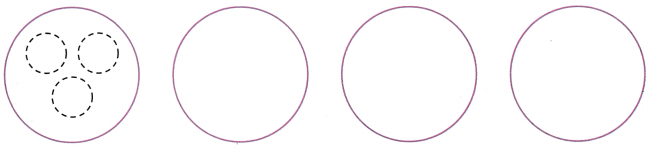Find the number of counters in all. Complete the addition sentence.
3 + _____ + _____ + _____ = _____
So, Tomeka needs _____ apples to make _____ cakes.
3 + 3 + 3 + 3 = 12
So, Tomeka needs 12 apples to make 4 cakes.Explanation:
Each counter has 3 apples.
4 counters has 12 apples.
Tomeka need 12 apples to make 4 cakes.

Math Talk
Mathematical Processes

Explain how the picture you drew shows groups of equal size.
Each cake is of same size and each cake needs 3 apples.
Total four cakes made up of 3 apples each, so total 12 apples are used to make 4 cakes.

Another Way Multiply.

When you combine equal groups, you can multiply to find how many in all.Draw 3 counters in each circle.Since there are the same number of counters in each circle, you can multiply to find how many in all.

Multiplication is another way to find how many there are altogether in equal groups.Read: Four times three equals twelve.
The factors are the numbers multiplied.
The product is the answer to a multiplication problem.

Share and Show

Question 1.
Write related addition and multiplication sentences for the model.5 + 5 + 5 + 5 = 20
5 x 4 = 20
Explanation:
Number of counters 4
Each counter has 5 circles
Total circles in each counters are 20

Math Talk
Mathematical Processes

How would you change this model so you could write a multiplication sentence to match it?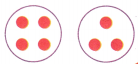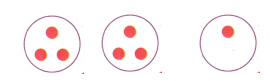Explanation:
Each counter has 4 circles
Distribute circles equally in each counter
3 x 2 + 1
6 + 1 = 7
Draw a quick picture to show the equal groups. Then write related addition and multiplication sentences.

Question 2.
3 groups of 6
_______ + _______ + _______ = _______
_______ × _______ = _______
6 + 6 + 6 = 18
6 × 3 = 18
Explanation:
Each counter is distributed into 3 groupsQuestion 3.
2 groups of 3
_______ + _______= _______
_______ × _______ = _______

3 + 3 = 6
2 × 3 = 6
Explanation:Each counter is distributed in 3 groups.
3 + 3 = 6
2 × 3 = 6

Problem Solving

Use the table for 4-5.Question 4.
Record Morris bought 4 peaches. How much do the peaches weigh in all? Write a multiplication sentence to find the weight of the peaches.
_______ × _______ = _______ ounces
weight of the peaches.
4 × 3 = 12 ounces
Explanation:
Morris bought 4 peaches.
One peach weighs 3 ounces.
Weight of 4 peaches = 4 × 3 = 12 ounces

Question 5.
H.O.T. Multi-Step Thomas bought 2 apples. Sydney bought 4 bananas. Which weighed more– -the 2 apples or the 4 bananas? How much more? Explain how you know.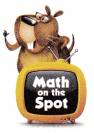4 bananas are more weight then 2 apples
Explanation:
weight of the apples.
2 × 6 = 12 ounces
weight of the bananas.
4 × 4 = 16 ounces

Question 6.
H.O.T. Write Math Write a word problem that can be solved using 3 × 4 Solve the problem.
If one banana is of 4 ounce, find the total weight of the 3 bananas?
Explanation:
If one banana is of 4 ounce,
weight of the 3 bananas.
3 × 4 = 12 ounces

Fill in the bubble for the correct answer choice.

Question 7.
Apply Laura and her mother make jam from berries and apples. When they are finished, they have 9 jars of apple jam and 9 jars of berry jam. Which multiplication sentence shows how many jars they made?
(A) 1 × 9 = 9
(B) 2 × 2 = 4
(C) 9 × 9 = 81
(D) 2 × 9 = 18
Option (D)
Explanation:
Apply Laura and her mother have 9 jars of apple jam and
9 jars of berry jam.
Number of jars they made
9 + 9 = 18
2 × 9 = 18

Question 8.
Billy has 3 stacks of books. Each stack has 6 books. Billy says that the number of books is equal to 6 + 6 + 6. Which is another way to find the number of books?
(A) 6 × 6 = 36
(B) 3 × 6 = 18
(C) 6 × 1 = 6 .
(D) 3 × 3 = 9
Option (B)
Explanation:
Billy has 3 stacks of books.
Each stack has 6 books.
Billy says that the number of books is equal to 6 + 6 + 6.
Another way to find the number of books
6 + 6 + 6 = 18
3 × 6 = 18

Question 9.
Multi-Step Carlos buys 4 packs of regular pencils. He buys 2 packs of color pencils. There are 4 pencils in each kind of pack. How many pencils did Carlos buy?
(A) 24
(B) 16
(C) 32
(D) 12
Option (A)
Explanation:
Carlos buys 4 packs of regular pencils.
Regular pencils = 4 × 4 = 16
He buys 2 packs of color pencils.
Color Pencils = 2 × 4 = 8
There are 4 pencils in each kind of pack.
Total pencils Carlos bought
16 + 8 = 24

Texas Test Prep

Question 10.
Which is another way to show 2 + 2 + 2 + 2?
(A) 2 × 2
(B) 5 × 2
(C) 4 × 2
(D) 2 × 8
Option (C)
Explanation:
2 + 2 + 2 + 2 = 8
Another way to show is multiplication
4 × 2 = 8

### Texas Go Math Grade 3 Lesson 6.1 Homework and Practice Answer Key

Draw a quick picture to show equal groups. Then write related addition and multiplication sentences.

Question 1.
3 groups of 4
_______ + _______ + _______ = _______
_______ × _______ = _______
4 + 4 + 4 = 12
3 × 4 = 12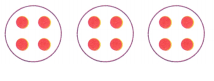Explanation:
Each counter has 4 groups
4 + 4 + 4 = 12
3 × 4 = 12

Question 2.
4 groups of 2
_______ + _______ + _______ + _______ = _______
_______ × _______ = _______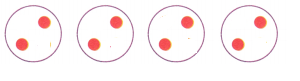2 + 2 + 2 + 2 = 8
2 × 4 = 8
Explanation:
Each counter has 2 groups
2 + 2 + 2 + 2 = 8
2 × 4 = 8

Use the table for 3-4.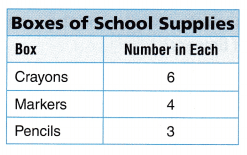Question 3.
Kaymer buys 2 boxes of crayons. Write a multiplication sentence to find how many crayons Kaymer buys.
_______ × _______ = _______
12 crayons
2 × 6 = 12
Explanation:
Kaymer buys 2 boxes of crayons.
Each box contains 6 crayons.
Total crayons in 2 boxes
2 x 6 = 12

Question 4.
Mya buys 4 boxes of pencils. Write a multiplication sentence to find how many pencils Mya buys.
_______ × _______ = _______
12 pencils
4 × 3 = 12
Explanation:
Mya buys 4 boxes of pencils.
Each box contains 3 pencils.
Total pencils in 4 boxes
4 x 3 = 12

Problem Solving

Question 5.
Zach buys 4 boxes of pens. Each pack has 4 pens. Write a multiplication sentence to show how many pens Zach buys.
_______ × _______ = _______
4 × 4 = 16
Explanation:
Zach buys 4 boxes of pens.
Each pack has 4 pens.
Total pens Zach bought
4 x 4 = 16

Question 6.
Ada has 3 vases. She puts 5 flowers in each vase. Write a multiplication sentence to show how many flowers Ada puts in the vases.
_______ × _______ = _______
3 × 5 = 15
Explanation:
Ada has 3 vases.
She puts 5 flowers in each vase.
Total flowers all in vases
3 x 5 = 15

Lesson Check

Question 7.
Jada makes 3 trays of muffins. Each tray holds 6 muffins. Which equation shows how many muffins Jada makes?
(A) 3 × 6 = 18
(B) 3 + 6 = 9
(C) 6 × 3 = 9
(D) 6 × 3 = 12
Option (A)
Explanation:
Jada makes 3 trays of muffins.
Each tray holds 6 muffins.
Total muffins Jada makes
3 × 6 = 18

Question 8.
Robinson puts 5 books on 2 shelves. Which equation shows how many books Robinson puts on the shelves?
(A) 2 + 5 = 10
(B) 5+2=7
(C) 5 × 2 = 10
(D) 2 × 5 = 7
Option (C)
Explanation:
Robinson puts 5 books on 2 shelves.
5 × 2 = 10

Question 9.
Midori puts 5 flowers in each of 3 vases. She says the number of flowers is equal to 5 + 5 + 5. Which is another way to find the number of flowers?
(A) 3 × 3
(B) 3 × 5
(C) 5 + 5
(D) 3 + 5
Option (B)
Explanation:
Midori puts 5 flowers in each of 3 vases.
She says the number of flowers is equal to 5 + 5 + 5.
Another way to find the number of flowers.
3 × 5 = 15

Question 10.
Pete has 5 boxes. Each box has 4 toy cars in it. Pete says the number of cars is equal to 4 + 4 + 4 + 4 + 4. Which is another way to find the number of cars?
(A) 5 + 4
(B) 4 × 4
(C) 5 × 5
(D) 5 × 4
Option (D)
Explanation:
Pete has 5 boxes.
Each box has 4 toy cars in it.
Pete says the number of cars is equal to 4 + 4 + 4 + 4 + 4.
Another way to find the number of cars
5 × 4 = 20

Question 11.
Multi-Step Mrs. Tomar buys 2 packs of vanilla yogurt and 3 packs of strawberry yogurt. Each pack has 4 yogurts. How many yogurts does Mrs. Tomar buy?
(A) 20
(B) 9
(C) 12
(D) 24
Option (A)
Explanation:
Mrs. Tomar buys 2 packs of vanilla yogurt and 3 packs of strawberry yogurt.
Each pack has 4 yogurts.
Total yogurts Mrs. Tomar bought
2 × 4 = 8
3 × 4 = 12
8 + 12 = 20

Question 12.
Multi-Step Murray buys 3 packs of red peppers and 4 packs of green peppers. Each pack has 4 peppers. How many peppers does Murray buy?
(A) 16
(B) 12
(C) 28
(D) 48